#SOLVING LOGARITHMIC EQUATIONS1. To solve a logarithmic equation, rewrite the equation in exponential form and solve for the variable.

Example 1: Solve for x in the equation Ln(x)=8.

Solution:

Step 1: Let both sides be exponents of the base e. The equation Ln(x)=8 can be rewritten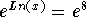.
Step 2: By now you should know that when the base of the exponent and the base of the logarithm are the same, the left side can be written x. The equationcan now be written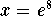.
Step 3: The exact answer is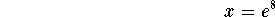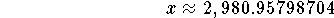Check: You can check your answer in two ways. You could graph the function Ln(x)-8 and see where it crosses the x-axis. If you are correct, the graph should cross the x-axis at the answer you derived algebraically.
You can also check your answer by substituting the value of x in the initial equation and determine whether the left side equals the right side. For example, if Ln(2,980.95798704)=8, you are correct. It does, and you are correct.

Example 2: Solve for x in the equation 7Log(3x)=15.

Solution:

Step 1: Isolate the logarithmic term before you convert the logarithmic equation to an exponential equation. Divide both sides of the original equation by 7: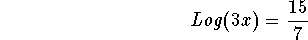Step 2: Convert the logarithmic equation to an exponential equation: If no base is indicated, it means the base of the logarithm is 10. Recall also that logarithms are exponents, so the exponent is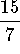. The equationcan now be written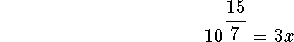Step 3: Divide both sides of the above equation by 3: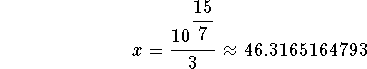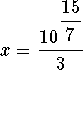is the exact answer and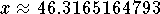is the approximate answer.

Check: You can check your answer in two ways: graphing the function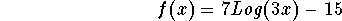or substituting the value of x into the original equation. If you choose graphing, the x-intercept should be the same as the answer you derived ().
If you choose substitution, the value of the left side of the original equation should equal the value of the right side of the equation after you have calculated the value of each side based on your answer for x.

Example 3: Solve for x in the equation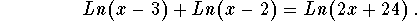Solution:

Step 1: Note the first term Ln(x-3) is valid only when x>3; the term Ln(x-2) is valid only when x>2; and the term Ln(2x+24) is valid only when x>-12. If we require that x be any real number greater than 3, all three terms will be valid. If all three terms are valid, then the equation is valid.
Step 2: Simplify the left side of the above equation: By the properties of logarithms, we know that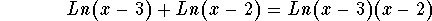Step 3: The equation can now be written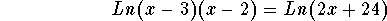Step 4: Let each side of the above equation be the exponent of the base e: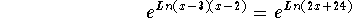Step 5: Simplify the above equation: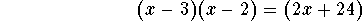Another way of looking at the equation in Step 3 is to realize that if Ln(a) = Ln(b), then a must equal b. In the case of this problem, thenStep 6: Simplify the left side of the above equation: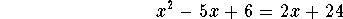Step 7: Subtract 2x + 24 from each side: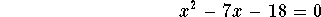Step 8: Factor the left side of the above equation: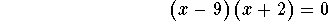Step 9: If the product of two factors equals zero, at least one of the factor has to be zero. If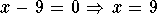. If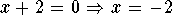. x = 9 is our only solution. Why is 9 the only solution? We defined our domain to be all the real numbers greater than 3.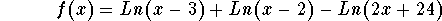and determining whether the x-intercept is also equal to 9. If it is, you have worked the problem correctly.
You could also check your answer by substituting 9 for x in the left and right sides of the original equation. If, after the substitution, the left side of the equation has the same value as the right side of the equation, you have worked the problem correctly.

If you would like to review another example, click on Example.

Work the following problems. If you wish to review the answer and the solution, click on Answer.

Problem 1: Solve for x in the equation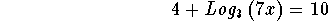Problem 2: Solve for x in the equation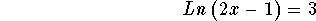Problem 3: Solve for x in the equation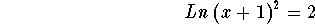Problem 4: Solve for x in the equation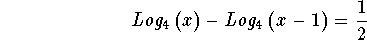Problem 5: Solve for x in the equation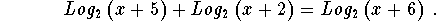Problem 6: Solve for x in the equation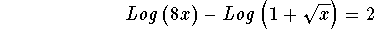[Back to Rules of Logarithms] [Back to Exponential Functions]

[Algebra] [Trigonometry] [Complex Variables]S.O.S MATHematics home page

Do you need more help? Please post your question on our S.O.S. Mathematics CyberBoard.Author: Nancy Marcus